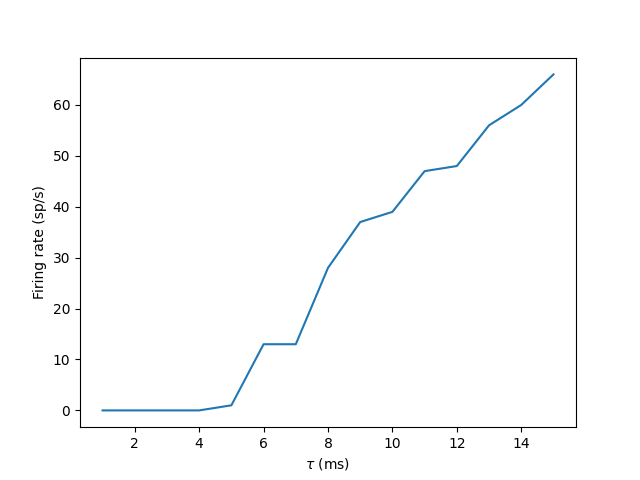# Example: standalone_multiplerun¶

Note

You can launch an interactive, editable version of this example without installing any local files using the Binder service (although note that at some times this may be slow or fail to open):This example shows how to run several, independent simulations in standalone mode. Note that this is not the optimal approach if running the same model with minor differences (as in this example).

The example come from Tutorial part 3. For a discussion see this post on the Brian forum.

import numpy as np
import pylab as plt
import brian2 as b2
from time import time

b2.set_device('cpp_standalone')

def simulate(tau):
# These two lines are needed to start a new standalone simulation:
b2.device.reinit()
b2.device.activate()

eqs = '''
dv/dt = -v/tau : 1
'''

net = b2.Network()
P = b2.PoissonGroup(num_inputs, rates=input_rate)
G = b2.NeuronGroup(1, eqs, threshold='v>1', reset='v=0', method='euler')
S = b2.Synapses(P, G, on_pre='v += weight')
S.connect()
M = b2.SpikeMonitor(G)

net.run(1000 * b2.ms)

return M

if __name__ == "__main__":
start_time = time()
num_inputs = 100
input_rate = 10 * b2.Hz
weight = 0.1
npoints = 15
tau_range = np.linspace(1, 15, npoints) * b2.ms

output_rates = np.zeros(npoints)
for ii in range(npoints):
tau_i = tau_range[ii]
M = simulate(tau_i)
output_rates[ii] = M.num_spikes / b2.second

print(f"Done in {time() - start_time}")

plt.plot(tau_range/b2.ms, output_rates)
plt.xlabel(r"$\tau$ (ms)")
plt.ylabel("Firing rate (sp/s)")
plt.show()